# Ham

This item is of food class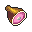You see a juicy ham.
Attributes
AMOUNT
80
FREQUENCY
10
REGEN
6
Dropped by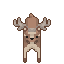deer
Drops between 1 and 2.
Probability: 30%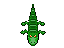caiman
Drops between 1 and 3.
Probability: 45%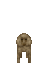monkey
Drops between 1 and 2.
Probability: 30%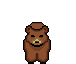bear
Drops between 1 and 3.
Probability: 30%lion
Drops between 1 and 2.
Probability: 30%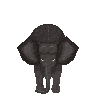elephant
Drops between 2 and 8.
Probability: 70%tiger
Drops between 1 and 2.
Probability: 30%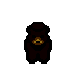black bear
Drops between 2 and 4.
Probability: 40%crocodile
Drops between 2 and 5.
Probability: 50%polar bear
Drops between 4 and 10.
Probability: 40%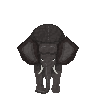bull elephant
Drops between 2 and 8.
Probability: 70%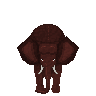musth elephant
Drops between 4 and 10.
Probability: 75%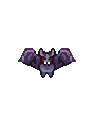giant bat
Drops between 3 and 5.
Probability: 5%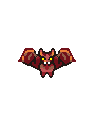giant killer bat
Drops between 5 and 8.
Probability: 5%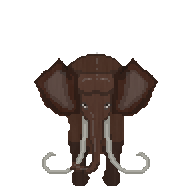woolly mammoth
Drops between 5 and 15.
Probability: 5%
Quests